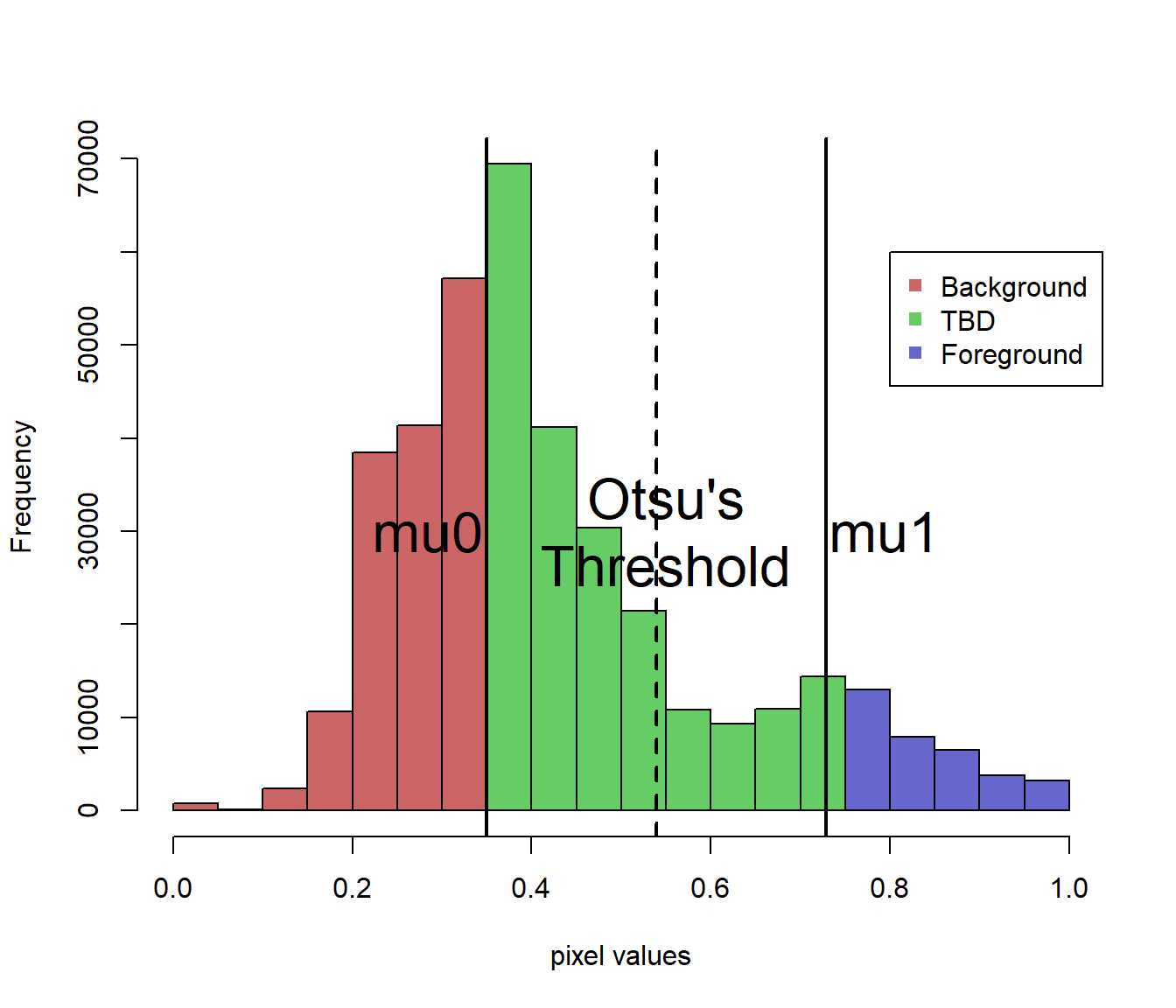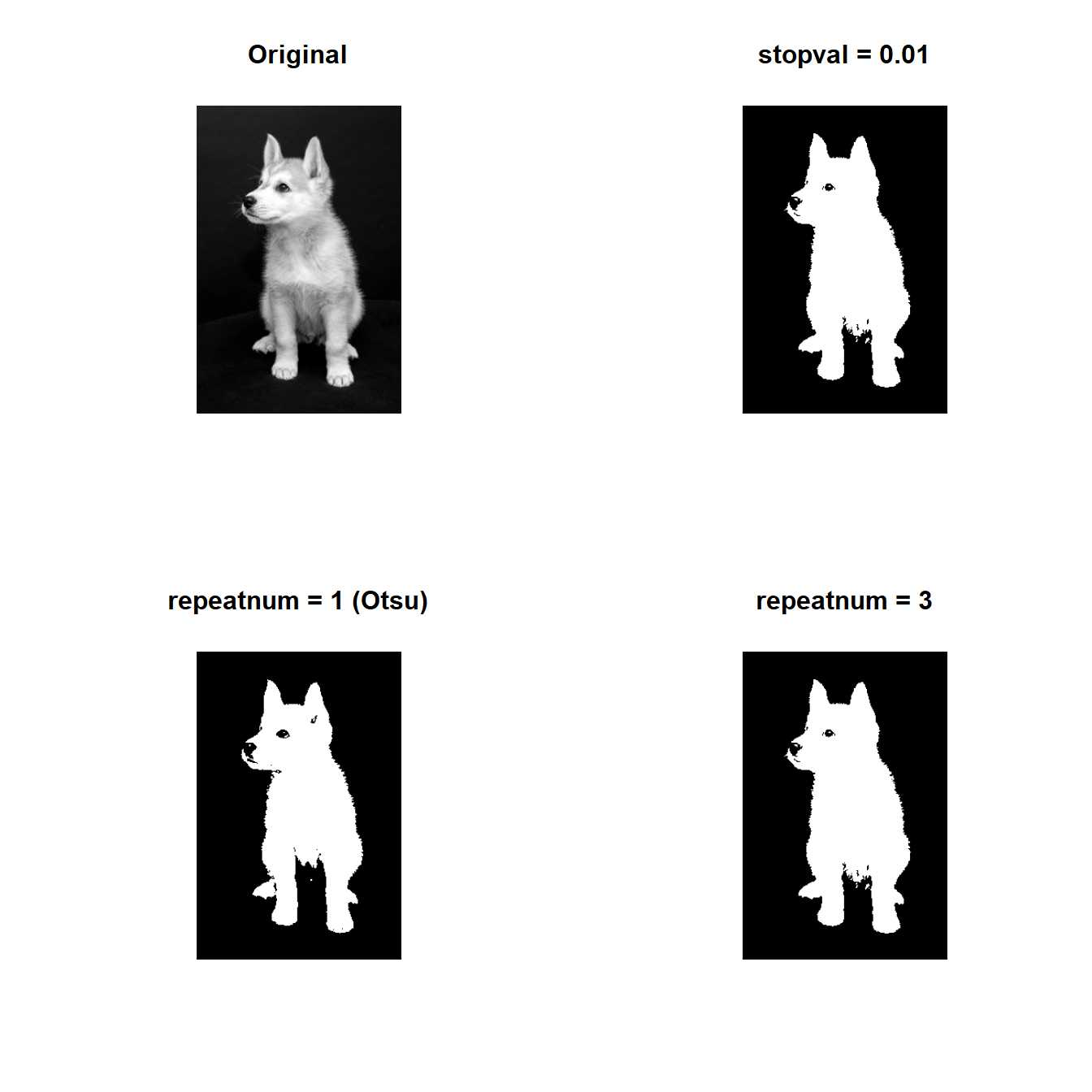Iterative Triclass Thresholding

Image Segmentation is a first process to analyze objects in image.

There are many segmenation algorithms.

One of them is Iterative Triclass Thresholding (ITT).

ITT is based on Otsu’s method but can be applied iteratively.

Otsu’s method divides pixels of an image into two classes, foreground and background, by a threshold.

ITT divides pixels into three classes: foreground, background, and “to-be-determined” (TBD).

Let’s see how ITT divides pixels into three classes.

First, we apply Otsu’s method on an image to get a threshold that divides pixels into two classes.

Then, compute the means of the two classes divided by the threshold.

($${\mu}_{1}$$: mean of foreground, $${\mu}_{0}$$: mean of background)

Finally, divide the pixles into three classes as below ($$u(x,y):$$ pixel value).

• Foreground: pixels whose values are larger than $${\mu}_{1}$$

$$u(x,y)>{\mu}_{1}$$

• Background: pixels whose values are smaller than $${\mu}_{0}$$

$$u(x,y)<{\mu}_{0}$$

• to-be-determined (TBD): pixels whose values are larger than or equal to $${\mu}_{0}$$ and smaller than or equal to $${\mu}_{1}$$

$${\mu}_{0}<=u(x,y)<={\mu}_{1}$$Figure 1: a histogram divided into three classes

Figure 1 shows a histogram divided into three classes.

Red means Background. Green means TBD. Blue means Foreground.

We can apply Otsu’s method on TBD region and divide TBD region into three classes.

Then, we have new TBD region again.

We can iterate division of TBD region.

After the end of iteration, we have a threshold value found by applying Otsu’s threshold on last TBD region.

The threshold is a threshold found by Iterative Triclass Threshold.

This iterative method is Iterative Triclass Thresholding.

When do we stop iteration?

We have two options.

• set preset threshold
• set repeat number

After each iteration (except for first iteration), we can compute the difference between new threshold and old threshold.

If the difference is smaller than preset threshold, we stop iteration.

This is the former way.

The latter way is needless to explain.

ThresholdTriclass() function performs Iterative Triclass Thresholding.

The argument ‘stopval’ controls preset threshold.

The argument ‘repeatnum’ controls repeat number.

Note that stopval is ignored if repeatnum is set.

library(devtools)
install_github("ShotaOchi/imagerExtra")
library(imagerExtra)
g <- grayscale(dogs)
layout(matrix(1:4, 2, 2, byrow = TRUE))
plot(g, main = "Original", axes=F)
ThresholdTriclass(g, stopval = 0.01) %>% plot(main = "stopval = 0.01", axes=F)
ThresholdTriclass(g, repeatnum = 1) %>% plot(main = "repeatnum = 1 (Otsu)", axes=F)
ThresholdTriclass(g, repeatnum = 3) %>% plot(main = "repeatnum = 3", axes=F)Figure 2: Results of Iterative Triclass Thresholding

We can see the belly of the dog was segmented as foreground after 3 times iteration.

The belly was not segmented as foreground by Otsu’s method.

ThresholdTriclass() perfoms Otsu’s method when repeatnum = 1.

This is one of characteristics of ITT.

ITT can overwhelm Otsu’s method in segmenting weak objects and fine details.

Reference: Cai HM, Yang Z, Cao XH, Xia WM, Xu XY (2014). A New Iterative Triclass Thresholding Technique in Image Segmentation. IEEE TRANSACTIONS ON IMAGE PROCESSING.